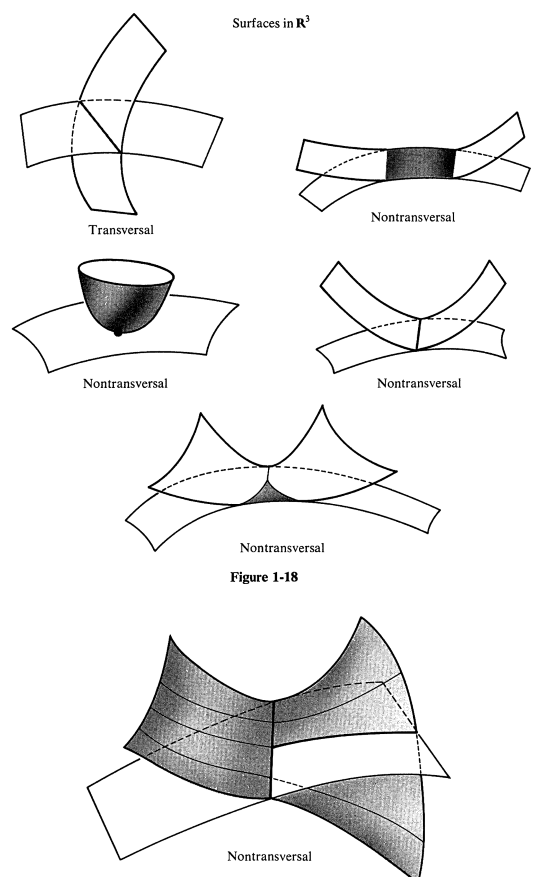Skip to main content

# MTH713A: Differential Topology

## Course Description

This is an introductory course in Differential Topology. The aim of this course is to introduce basic tools to study the topology and geometry of manifolds. We start with reviewing two key results from several variable calculus, namely the inverse function theorem and implicit function theorem which are essential to study differential manifolds. Throughout this course, we will discuss the theory of manifolds and a way to generalise differential, integral and vector calculus. By the end of the course, we should understand and able to work with manifolds, tangent and co-tangent bundles, transversality, Morse Lemma, Morse function, Whitney embedding theorem, Poincare – Hopf theorem, Sards theorem and its applications and many other things listed below in the course contents.Transver/Nontransversal intersection

## Course Content

• Review of inverse function theorem and implicit function theorem.
• Introduction to differential manifolds, sub-manifolds and manifolds with boundary.
• Smooth maps between differential manifolds, tangent space, differential of smooth maps,
• (local) diffeomorphism. Immersions, embeddings, regular value, level sets, submersions.
• Tangent and cotangent bundles, vector bundles, vector fields, integral curves.
• Transversality, Whitney embedding theorem, Sards theorem, Morse lemma, Morse functions.
• Oriented intersection Theory: degree, Lefchetz fixed point theory, the Poincare-Hopf theorem,
• Euler characteristic and triangulations (time permitting: Integration on manifolds).

References.

1. Differential Topology, Victor Guillemin and Alan Pollack (AMS Chelsea Book Series)

2. Spivak: Calculus on manifolds, Springer.

3. Topics in Differential Topology, Amiya Mukherjee (Hindustan Book Agency)

4. Topology from the Differentiable Viewpoint, John W. Milnor (Princeton University Press)

5. An Introduction to Differentiable Manifolds and Riemannian Geometry, William M.
Boothby (Elsevier)

6. An introduction to manifolds, Loring W Tu (Springer).

## Course Audience

Those (MSc 2nd year and PhD students) who are interested in topology and geometry, have done the courses Topology and Several variables calculus and differential geometry.